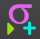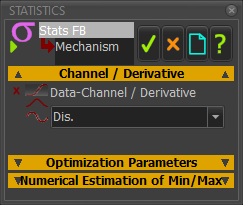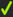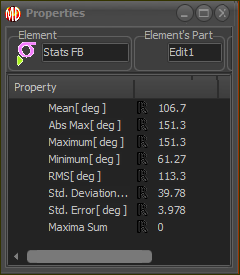﻿ MechDesigner Reference & User Interface > Dialog-Boxes > Dialog: Function-Block: Statistics

# Dialog: Function-Block: Statistics

Navigation:  MechDesigner Reference & User Interface > Dialog-Boxes >

# Dialog: Function-Block: Statistics

## Statistics FB

### Statistics FB

If you can connect a wire to a Graph FB to plot its data over a complete machine-cycle ...

... then you can connect a wire to a Statistics FB to find statistical data for the complete machine-cycle.

Compare with Element Properties dialog, which displays the data-values at the only.

There are two steps you need to do to get the Statistics for a Data-Channel on a wire.

STEP 0: Connect a wire to the input of the Statistics FB.

A wire that you connect from the output-connector of a FB to the input-connector of the Statistics FB has three Data-Channels.

For example, the three Data-Channels at the output-connector of a Motion-Dimension FB are the three motion-derivatives of a dimension

STEP 1: Select the Data-ChannelSTEP 1.Open the Statistics dialog-box

Edit the Statistics FB:

 1.Double-click the Statistics FB in the graphic-area - or - 1.

The Statistics FB dialog-box is now open.Channel / Derivative

STEP 2.Click the Derivative drop-down and select the derivative for which you want Statistics

 Derivative ≡ Data-Channel Dis. Linear Position or Angular Angle or Torque Vel. Linear Velocity or Angular Velocity or Force X Acc. Linear Acceleration or Angular Acceleration or Force Y

* The Data-Channels in the drop-down box are always: Dis, Vel, and Acc , even if the actual units are different. The actual units:

For a Kinematic-FB are three(3) motion-derivatives

For a Force-Data FB are Total Force/Torque, Force X, Force Y.

Ignore the other separators in the dialog.

STEP 3.Clickto close the Statistics dialog-box

STEP 2: Show the Statistics PropertiesStatistic Properties dialog

STEP 1.Open the Properties box

 1.CTRL + Click the Statistics FB in the graphic-area

The Properties box is now open.

Read the Statistical Properties for the Data-Channel of the wire that is connected to its input-connector.

Mean

Ans Max

Maximum

Minimum

RMS

Standard Deviation

Standard Error

Maximum Sum

Note: to edit the Number-Format for the Statistics Properties, use:

Application-Settings > Number-Format > Data-Output Format > Digits and Precision